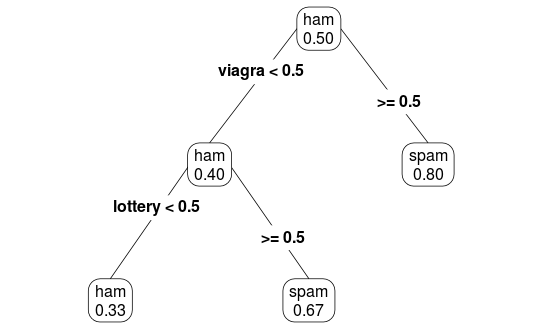# Growing a Spam Tree

· Big Data Zone · Interview
Save
2.35K Views

consider the following toy dataset , with some spam/ham information, and two words, “viagra” and “lottery”.

``````> load(spam.rdata)
> head(db)
y viagra lottery
27 spam      0       1
37  ham      0       1
57 spam      0       0
89  ham      0       0
20 spam      1       0
86  ham      0       0``````

for the first node, compute gini index for the two variables,

``````> gini=function(variable){
+ t=table(db\$y,db[,variable])
+ nx=apply(t,2,sum)
+ probcond=t/matrix(rep(nx,each=2),2,2)
+ probcond
+ gini=-probcond*(1-probcond)
+ sum(matrix(rep(nx,each=2),2,2)/sum(nx)*gini)}
> gini("viagra")
 -0.44
> gini("lottery")
 -0.487``````

here the gini index is maximal for “viagra”, so that will be the first node.

on the left node (emails without “viagra”), the component of gini index is

``````> -75/100*(.4*.6+.6*.4)
 -0.36``````

if we decide to split (using the second word, “lottery”), at this node, the new gini index would be

``````> idx=which(db\$viagra==0)
> t=table(db[idx,"y"],db[idx,"lottery"])
> nx=apply(t,2,sum)
> probcond=t/matrix(rep(nx,each=2),2,2)
> gini=-probcond*(1-probcond)
> sum(matrix(rep(nx,each=2),2,2)/100*
+       gini)
 -0.333``````

since gini index is larger, we decide to split (based on the second word) here. on the other node (emails with “viagra”), the component of gini index is

``````> -25/100*(.8*.2+.2*.8)
 -0.08``````

and if decide to split (again, according to the second word), we get

``````> idx=which(db\$viagra==1)
> t=table(db[idx,"y"],db[idx,"lottery"])
> nx=apply(t,2,sum)
> probcond=t/matrix(rep(nx,each=2),2,2)
> gini=-probcond*(1-probcond)
> sum(matrix(rep(nx,each=2),2,2)/100*
+       gini)
 -0.0792``````

which is only slightly larger. splitting would not be very interesting, here. to visualize the tree, use

``````> library(rpart)
> arbre = rpart(factor(y)~.,data=db)
> library(rpart.plot)
> rpart.plot(arbre,type=4,extra=6)``````Topics:
bigdata, big data, data visualization

Published at DZone with permission of Arthur Charpentier, DZone MVB.

Opinions expressed by DZone contributors are their own.

Comments